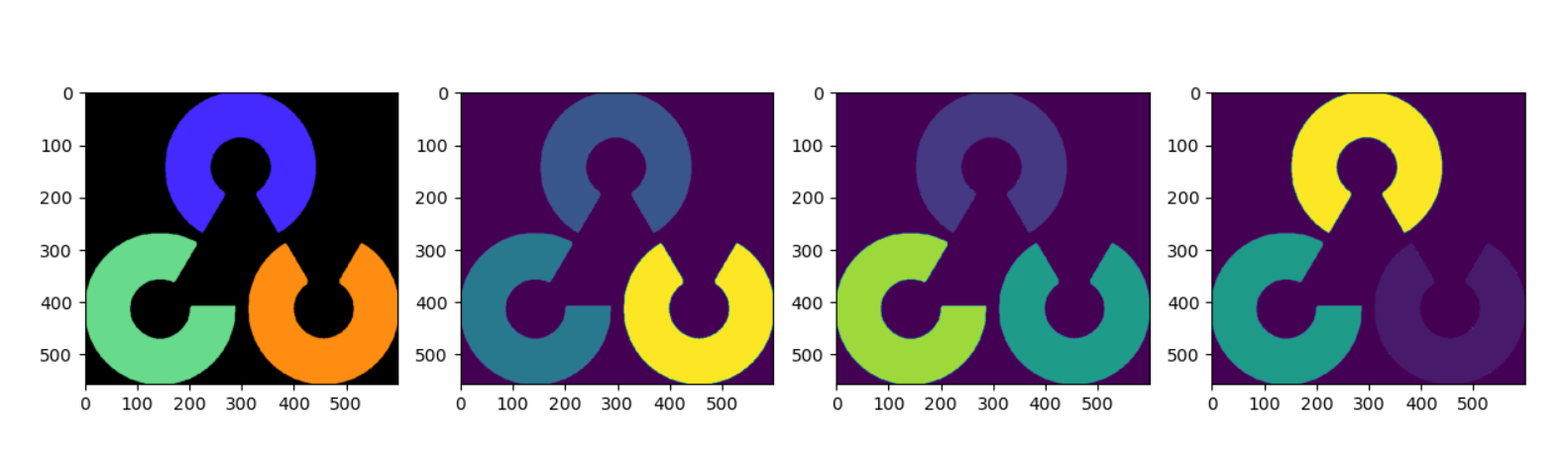# 1、如何證明OpenCV讀取的通道是BGR通道？

```cpp import matplotlib.pyplot as plt import cv2 import numpy as np img=cv2.imread('D://00.png') b,g,r = cv2.split(img) cv2.namedWindow("original_img", cv2.WINDOW_NORMAL) cv2.imshow("original_img",img) cv2.namedWindow("img_b", cv2.WINDOW_NORMAL) cv2.imshow("img_b",b) cv2.namedWindow("img_g", cv2.WINDOW_NORMAL) cv2.imshow("img_g",g) cv2.namedWindow("img_r", cv2.WINDOW_NORMAL) cv2.imshow("img_r",r) cv2.waitKey(0)``` 步驟：

C++版代碼： ```cpp

# include

using namespace cv; int main() { Mat src = imread("00.png"); namedWindow("src", CV_WINDOW_NORMAL); imshow("src", src);//原圖像 Mat rgb; split(src, rgb); namedWindow("B", CV_WINDOW_NORMAL); imshow("B", rgb);//第一個通道存放B分量 namedWindow("G", CV_WINDOW_NORMAL); imshow("G", rgb);//第二個通道存放G分量 namedWindow("R", CV_WINDOW_NORMAL); imshow("R", rgb);//第三個通道存放R分量 waitKey(0); } ``` 使用plt做圖片展示

```python import matplotlib.pyplot as plt import cv2 import numpy as np img=cv2.imread('D://00.png') b,g,r = cv2.split(img) fig, axes = plt.subplots(1, 4, figsize=(15, 15)) axes.imshow(img) axes.imshow(b) axes.imshow(g) axes.imshow(r) plt.show()``` 輸出圖片：plt展示的原始圖片，我們就可以看出BGR通道了。

# 2、如何證明使用imdecode將解碼的圖片轉為BGR圖片。

imdecode方法中有這樣一句話，在彩色圖像的情況下，解碼後的圖像將以 B G R 順序存儲通道。我用下面的代碼證明這句話。 代碼： ```python with open("D://00.png", "rb") as image: f = image.read() # convert to numpy array image = np.asarray(bytearray(f)) img = cv2.imdecode(image, 1) b, g, r = cv2.split(img) cv2.namedWindow("original_img", cv2.WINDOW_NORMAL) cv2.imshow("original_img", img) cv2.namedWindow("img_b", cv2.WINDOW_NORMAL) cv2.imshow("img_b", b) cv2.namedWindow("img_g", cv2.WINDOW_NORMAL) cv2.imshow("img_g", g) cv2.namedWindow("img_r", cv2.WINDOW_NORMAL) cv2.imshow("img_r", r) cv2.waitKey(0)``` 輸出結果：